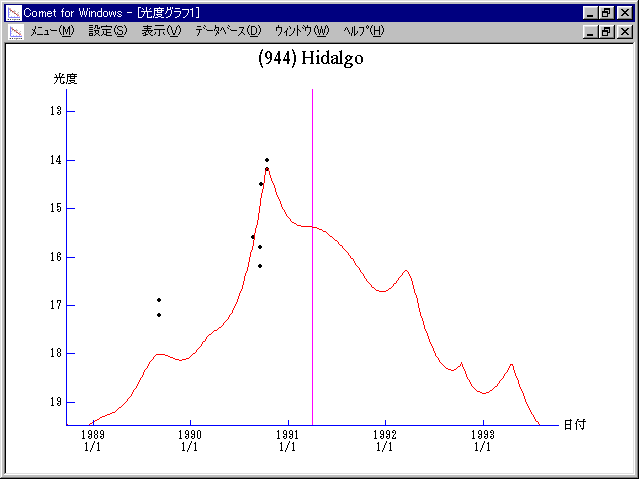# \$B>.OG@1%\$%@%k%4(B

(944) Hidalgo (1991)###\$B50F;MWAG(B

```   The following improved orbital elements by Kenji Muraoka, are
from 208 observations 1920 to 1999, perturbations by 9 Plantes,
Moon and 5 minor planets were taken into account.
The mean residual is +/- 1.02 arc seconds.

T  =  1991 Apr.  1.59579  TT    Epoch  =  1991 Apr. 14.0  TT
Peri. =   57.35248                     e  =    0.6574060
Node  =   21.69222    (2000.0)         a  =    5.8368599 AU
Incl. =   42.41988                     n\$B!,(B =    0.069893239
q  =    1.9996732 AU                P  =   14.102 years
```

###\$B@1?^(B1990\$BG/(B 1\$B7n(B 3\$BF|!A(B1991\$BG/(B 6\$B7n(B27\$BF|(B1991\$BG/(B 4\$B7n(B 8\$BF|!A(B1993\$BG/(B 8\$B7n(B15\$BF|(B

###\$B8wEYJQ2=(B

```        H = 10.8  G = 0.15
```##### \$B50F;MWAG\$OB<2,7r<#;a\$N7W;;\$K\$h\$k\$b\$N\$G\$9!#(B \$B@1?^\$O(B StellaNavigator Ver.2.0 for Windows (\$B%"%9%H%m%"!<%D(B \$BJTCx(B / \$B%"%9%-!<=PHG6I4)(B) \$B\$G:n@.\$7\$?\$b\$N\$G\$9!#(B \$B8wEY%0%i%U\$O(BComet for Windows\$B\$G:n@.\$7\$?\$b\$N\$G\$9!#(B